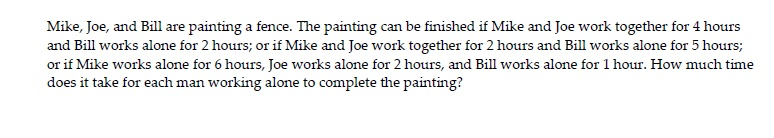Mike, Joe, and Bill are painting a fence. The painting can be finished if Mike and Joe work together for 4 hoursand Bill works alone for 2 hours; or if Mike and Joe work together for 2 hours and Bill works alone for 5 hours;or if Mike works alone for 6 hours, Joe works alone for 2 hours, and Bill works alone for 1 hour. How much timedoes it take for each man working alone to complete the painting?

Question

Solve the problem.help_outlineImage TranscriptioncloseMike, Joe, and Bill are painting a fence. The painting can be finished if Mike and Joe work together for 4 hours and Bill works alone for 2 hours; or if Mike and Joe work together for 2 hours and Bill works alone for 5 hours; or if Mike works alone for 6 hours, Joe works alone for 2 hours, and Bill works alone for 1 hour. How much time does it take for each man working alone to complete the painting? fullscreen
Step 1

Let M, J, B = fences per hour for Mike, Joe, Bill respectively.

Equations:

1. 4 (M + J) + 2 B = 1 (i)
2. 2 (M + J) + 5 B = 1    (ii)
3. 6 M + 2 J + 1 B = 1   (iii)

Let's Franklin be the person can work as fast as Mike and Joe together.

So, F = M + J
From the first equation, we get
4 F + 2 B = 1 fence
From the second equation, we get
2 F + 5 B = 1 or
4 F + 10 B = 2

Subtracting equation (i) from equation (ii) , we get
8 B = 1
B = 1/8
So, Bill paints the fence in 8 hours.

Step 2

From equation (iii) we have
6 M + 2 J + 1/8 = 1
6 M + 2 J = 7/8            (iv)

From equation (ii) we have
2 M + 2 J = 3/8            (v)
Subtracting equation (iv) f...

Want to see the full answer?

See Solution

Want to see this answer and more?

Our solutions are written by experts, many with advanced degrees, and available 24/7

See Solution
Tagged in

Algebra